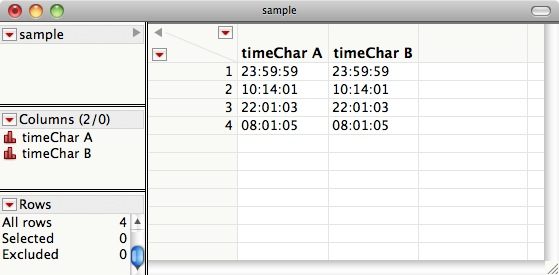## How to change string columns to numeric date columns

by on Jul.22, 2010 , under JMP & JSL

Sudarshan Krishnan asked a question on the LinkedIn JMP Professional Network about how to change a string column into a numeric date column:

What is the JSL command to convert a character data type (“hr:m:s” –eg:”23:59:59″) to a Numeric, Continuous, duration (“hr:m:s”) data type?

## Dates and times in a character column

Let’s assume you have a data table includes date, time, or duration values as strings, in character columns. You might run into this situation frequently when importing data from text files or from other programs such as Microsoft Excel. The columns might look like this:Here’s a simple JSL script you can run to create a data table like this one:

```New Table( "sample",
New Column( "timeChar A",
Character,
Nominal,
Set Values( {"23:59:59", "10:14:01", "22:01:03", "08:01:05"} )
),
New Column( "timeChar B",
Character,
Nominal,
Set Values( {"23:59:59", "10:14:01", "22:01:03", "08:01:05"} )
)
);```

## Convert date/time strings to numeric

You have two options for how to handle this, depending on what your goals are. You can either make a new, numeric column that converts the strings to date/time values using a formula, or you can change the character column directly into a numeric column. Let’s try it each way.

### Option A: make a new numeric column

To make a new numeric copy of the original column, use the `New Column()` operator with the arguments specifying the new column’s name (“timeNum A”), data type (Numeric), the new format desired (in this case “hr:m:s”), and a formula. The formula is simply the original column, :timeChar A, inside the `Num()` operator.

```New Column( "timeNum A",
Numeric,
Format( "hr:m:s" ),
Formula( Num( :timeChar A ) )
);```

Now you have the original string column, unchanged, plus a new numeric copy of that column. Note that the new column is linked to the original with a formula, so if you want to delete the original column, you’ll get an error:

Cannot delete column “timeChar A” while it is referenced by the formula of column “timeNum A”. Removing a formula leaves the data unchanged. Removing references replaces each reference with an empty value. These effects are permanent and cannot be undone.

Just click “Remove Formula” to proceed. Of course, if you no longer want the original character column, you might as well go with Option B instead.

### Option B: change a character column to numeric directly

To change an existing character column into numeric, just send a series of messages to the column, first changing the type to numeric, then specifying a format. You might also want to change the modeling type to continuous, and you might want to change the column’s name.

```Column( "timeChar B" )
<< data type( numeric )
<< Format( "hr:m:s" )
<< modeling type( continuous )
<< set name( "timeNum B" );```

## After

Now the data table with two character columns should have one character column, a second column that was character and has been changed to numeric, and a third column that is a numeric copy of the first column: